• 合并排序与插入排序比较 实验题目 合并排序与插入排序比较 实验要求 画出运行时间与n变化曲线对比图，并分析原因 实验目的 1、 掌握合并排序与插入排序的算法思想 2、 编写实现合并排序与插入排序算法 3、 比较合并...
(python)合并排序与插入排序比较实验
实验题目
合并排序与插入排序比较实验
实验要求
画出运行时间与n变化曲线对比图，并分析原因
实验目的
1、	掌握合并排序与插入排序的算法思想
2、	编写实现合并排序与插入排序算法
3、	比较合并排序与插入排序不同n值所耗费的时间
实验步骤
1、非递归实现插入排序算法
以下面5个无序的数据为例：65 27 59 64 58
第1次插入: 27 65 59 64 58
第2次插入: 27 59 65 64 58
第3次插入: 27 59 64 65 58
第4次插入: 27 58 59 64 65
#非递归实现插入排序
def Insert_sort(list):
for i in range(1, len(list)):
j = i
while j>0 and list[j-1]>list[j]:
list[j-1], list[j] = list[j], list[j-1]
j -= 1

2、合并排序算法实现
同样以下面5个无序的数据为例：65 27 59 64 58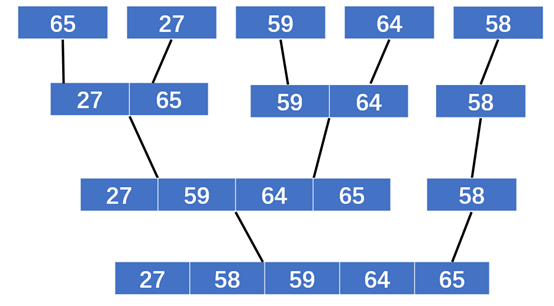#合并排序
def merge(a, b):
c = []
h = j = 0
while j < len(a) and h < len(b):
if a[j] < b[h]:
c.append(a[j])
j += 1
else:
c.append(b[h])
h += 1
if j == len(a):
for i in b[h:]:
c.append(i)
else:
for i in a[j:]:
c.append(i)
return c

def Merge_sort(list):
if len(list) <= 1:
return list
middle = len(list)//2
left = Merge_sort(list[:middle])
right = Merge_sort(list[middle:])
return merge(left, right)

3、统计函数运行时间
#函数运算时间
def time_Counter(func, seq):
time_start = time.time()
func(seq)
time_end = time.time()
return time_end-time_start

4、可视化显示实验结果
def ShowTimes(N,time1, time2):
#设置汉字格式
font = FontProperties(fname=r"c:\windows\fonts\simsun.ttc", size=14)
plt.figure("Lab3")
plt.title(u'不同n值插入排序与合并排序算法所耗费的时间',FontProperties=font)
plt.xlabel(u'n值',FontProperties=font)
plt.ylabel(u'所需时间(s)',FontProperties=font)

plt.scatter(x=N, y=time1, color='black',s=10)
plt.scatter(x=N, y=time2, color='red',s=10)

plt.plot(N,time1,color='black')
plt.plot(N,time2,color='red')

insert_sort = mlines.Line2D([], [], color='black', marker='.',
markersize=10, label='insert_sort')
Merge_sort = mlines.Line2D([], [], color='red', marker='.',
markersize=10, label='Merge_sort')
plt.legend(handles=[insert_sort,Merge_sort])
plt.show()


5、主函数设计
if __name__ == '__main__':
N = []
time1 = []
time2 = []
for i in range(10,5000,100):
list = []
for j in range(0,i+1):
list.append(j)
random.shuffle(list)
#复制列表list,保证两个排序函数进行排序的是同样的列表
list_copy = list[:]
#插入排序所耗费的时间
time1.append(time_Counter(Insert_sort,list))
#合并排序所耗费的时间
time2.append(time_Counter(Merge_sort, list_copy))
N.append(i)
ShowTimes(N,time1,time2)
#测试两个排序算法是否排序正确
test = []
for j in range(0,20):
test.append(j)
random.shuffle(test)
test_copy = test[:]
print('原数据为：',test)
Insert_sort(test)
print('插入排序后：',test)
print('合并排序后：',Merge_sort(test_copy))
print('插入排序所耗时间：',time1)
print('合并排序所耗时间：',time2)

6、输出显示结果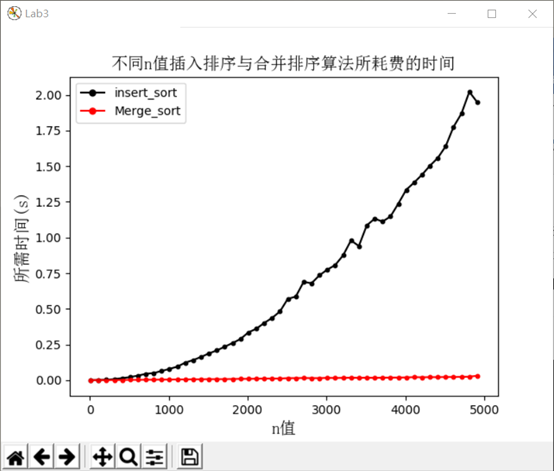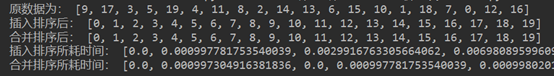全部实验代码
import time
import random
import sys
sys.setrecursionlimit(1000000)
from matplotlib.font_manager import FontProperties
import matplotlib
matplotlib.use('TkAgg')
import matplotlib.lines as mlines
import matplotlib.pyplot as plt

#非递归实现插入排序
def Insert_sort(list):
for i in range(1, len(list)):
j = i
while j>0 and list[j-1]>list[j]:
list[j-1], list[j] = list[j], list[j-1]
j -= 1
#合并排序
def merge(a, b):
c = []
h = j = 0
while j < len(a) and h < len(b):
if a[j] < b[h]:
c.append(a[j])
j += 1
else:
c.append(b[h])
h += 1
if j == len(a):
for i in b[h:]:
c.append(i)
else:
for i in a[j:]:
c.append(i)
return c

def Merge_sort(list):
if len(list) <= 1:
return list
middle = len(list)//2
left = Merge_sort(list[:middle])
right = Merge_sort(list[middle:])
return merge(left, right)

#函数运算时间
def time_Counter(func, seq):
time_start = time.time()
func(seq)
time_end = time.time()
return time_end-time_start

def ShowTimes(N,time1, time2):
#设置汉字格式
font = FontProperties(fname=r"c:\windows\fonts\simsun.ttc", size=14)
plt.figure("Lab3")
plt.title(u'不同n值插入排序与合并排序算法所耗费的时间',FontProperties=font)
plt.xlabel(u'n值',FontProperties=font)
plt.ylabel(u'所需时间(s)',FontProperties=font)

plt.scatter(x=N, y=time1, color='black',s=10)
plt.scatter(x=N, y=time2, color='red',s=10)

plt.plot(N,time1,color='black')
plt.plot(N,time2,color='red')

insert_sort = mlines.Line2D([], [], color='black', marker='.',
markersize=10, label='insert_sort')
Merge_sort = mlines.Line2D([], [], color='red', marker='.',
markersize=10, label='Merge_sort')
plt.legend(handles=[insert_sort,Merge_sort])
plt.show()

if __name__ == '__main__':
N = []
time1 = []
time2 = []

for i in range(10,5000,100):
list = []
for j in range(0,i+1):
list.append(j)
random.shuffle(list)
#复制列表list,保证两个排序函数进行排序的是同样的列表
list_copy = list[:]
#插入排序所耗费的时间
time1.append(time_Counter(Insert_sort,list))

#合并排序所耗费的时间
time2.append(time_Counter(Merge_sort, list_copy))
N.append(i)
ShowTimes(N,time1,time2)
#测试两个排序算法是否排序正确
test = []
for j in range(0,20):
test.append(j)
random.shuffle(test)
test_copy = test[:]
print('原数据为：',test)
Insert_sort(test)
print('插入排序后：',test)
print('合并排序后：',Merge_sort(test_copy))
print('插入排序所耗时间：',time1)
print('合并排序所耗时间：',time2)




展开全文算法
• merge sort 主要运用了递归调用的思想，这种排序需要将 list 对半拆分到最小单位，即长度小于等于1，并将最小单位的list分别返回给 left 和 right 。然后通过 merge_sort 函数对其进行排序，将排好序的 list 再进行...
merge sort 主要运用了递归调用的思想，这种排序需要将 list 对半拆分到最小单位，即长度小于等于1，并将最小单位的list分别返回给 left 和 right 。然后通过 merge_sort 函数对其进行排序，将排好序的 list 再进行返回。通过对函数的反复调用，最终实现对 list 的排序。
def merge_split_sort(nums: list) -> list: # 拆分 + 合并
if len(nums) <= 1:
return nums
m = len(nums) // 2
left = merge_split_sort(nums[:m]) # 递归调用
right = merge_split_sort(nums[m:])
return merge_sort(left, right)

def merge_sort(left: list, right: list) -> list: # 排序
c = []
while len(left) > 0 and len(right) > 0:
if left < right:
c.append(left)
left.remove(left)
else:
c.append(right)
right.remove(right)
if len(left) == 0:
c = c + right
else:
c = c + left
return c

def merge_sorted(nums: list, reverse = False):
nums_copy = merge_split_sort(nums)
if reverse:
nums_copy = nums_copy[::-1]
return nums_copy

L = [1, 3, 5, 7, 9, 2, 4, 6, 8, 0] # list for test
L = merge_sorted(L, True)
print(L)



展开全文算法
• python实现递归算法 一、开发环境 开发工具：jupyter notebook 并使用vscode，cmd命令行工具协助编程测试算法 编程语言：python3.6 二、实验内容 问题1，实现 fibonacci 的递归和非递归。要求计算F（100）的值，...
python实现递归算法

一、开发环境

开发工具：jupyter notebook 并使用vscode，cmd命令行工具协助编程测试算法
编程语言：python3.6

二、实验内容

问题1，实现 fibonacci 的递归和非递归。要求计算F（100）的值，比较两种方法的性能要求1）有合适的提示从键盘输入数据；例如“Please input the number to calculate:”2）有输出结果（下同）

Fibonacci非常有名，这里就不多介绍了，直接实现代码。

非递归版本实现的fibonacci数列代码：

# 实现fibonacci的递归算法以及非递归算法
# fibonacco数列前几项 为了检验结果是否正确 1 1 2 3 5 8 13 21 34

# 使用非递归建立fibonacci数列
def fibonacci(n):
a = 1
b = 1
if n==1 or n==2:p
return 1
else:
for i in range(n-2):
a, b = b, a+b
return b

if __name__ == '__main__':
import time
n = int(input("Please input the number to calculate:"))
start = time.time()
print(fibonacci(n))
end = time.time()
print("非递归版本的运行时间为", end-start)


利用jupyter notebook的运行工具进行运行，运行的出的结果为：递归版本实现的fibonacci数列代码（效率低下版本）

# 低效的做法
def fibonacci_recursion(n):
if n<=2:
return 1
else:
return fibonacci_recursion(n-1)+fibonacci_recursion(n-2)


这个版本的代码实现起来非常容易，并且十分易懂，不过缺点就是效率实在是太低了，当输入100的时候，计算了相当长的时间，所以考虑换一种思路，按照非递归版本的方法，从前依推导出后面的数据。

递归版本实现的fibonacci数列代码（换思路的版本）

# 使用递归建立fibonacci数列
def fibonacci_recursion(n, a=1, b=1, c=3):
if n < 3:
return 1
else:
if n==c:
return a+b
else:
return fibonacci_recursion(n, a=b, b=a+b, c=c+1)

if __name__ == '__main__':
import time
n = int(input("Please input the number to calculate:"))
start = time.time()
print(fibonacci_recursion(n))
end = time.time()
print("递归版本的运行时间为", end-start)


这一版本的代码虽然比起上面的复杂了点，但是效率还是非常快的。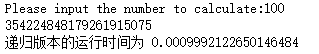EXTRA

上面的版本可以说是挺好的了，然而当我输入2000的时候，还是报错了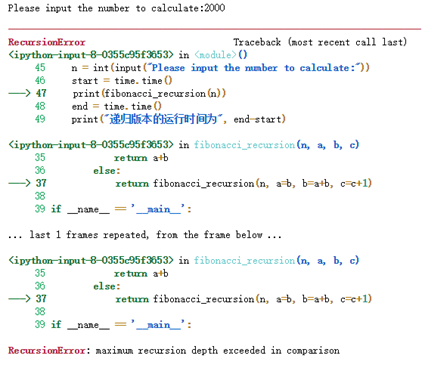好像说是超过了最大递归的深度，爆栈了。

依稀地记得，以前写代码的时候有过这种问题的解决方案，于是开始百度。

然而尴尬的是找了半天，并没有找到我上次的那份代码，上次的好像是用上了装饰器解决问的，不过查到了另外的解决方案，使用尾递归优化，并使用了python的生成器机制。代码如下：

# python 使用尾递归优化
import types    # 导入types模块
# 如果函数中出现了yield解释器会自动将函数当成生成器，此时是不会直接生成数的
# tramp的作用为：使生成器不断产生下一个数据
def tramp(gen, *args, **kwargs):
g = gen(*args, **kwargs)
while isinstance(g, types.GeneratorType):   # 如果g为生成器的实例
g=g.__next__()  #
return g

def fibonacci_recursion(n, a=1, b=1, c=3):
if n < 3:
yield 1
else:
if n==c:
yield a+b
else:
yield fibonacci_recursion(n, a=b, b=a+b, c=c+1)

if __name__ == '__main__':
import time
n = int(input("Please input the number to calculate:"))
start = time.time()
print(fibonacci_recursion(n))
end = time.time()
print("递归版本的运行时间为", end-start)


网上找到的代码是py2的版本的，最近都是用的py3，版本问题还真是头疼呀，花了点时间改了一下。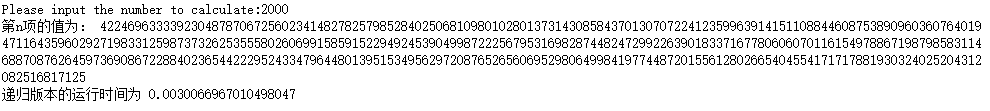经过优化的递归函数效果惊人,2000以内的都不在是问题啦。

问题2,实现全排列算法

实现前20个奇数的全排列。
实现数字1，3，5，7，9五个数字的全排列
实现对数字1,3,5,7,9五个数字的全排列

# 实现全排列的算法。
# a) 实现数字1，3，5，7，9五个数字的全排列。
# b) 实现前20个奇数的全排列。

# 全排列函数
def full_arrangement(lis):
length = len(lis)
if length < 2:    # 边界条件
return lis
else:
result = []
for i in range(length):
ch = lis[i]    # 取出str中每一个字符
rest = lis[0: i] + lis[i+1: length]
for s in full_arrangement(rest):  # 递归
result.append(ch+s) # 将ch与子问题的解依次组合
return result

if __name__ == '__main__':
lis = [1, 3, 5, 7, 9]
lis1 = list(map(str, lis))

import time
start = time.time()
print(full_arrangement(lis1))
end = time.time()
print("全排列运行时间：", end-start)


对五个数进行全排列的运行时间：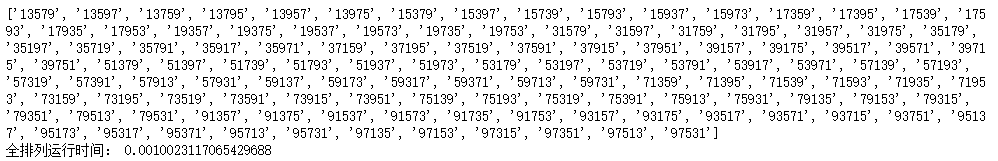下一个问题：实现前20个奇数的全排列。

测试代码已经写好了，是这个样子的

lis2 = list(map(str, [x for x in range(40) if x%2!=0]))
print(lis2)
start = time.time()
print(full_arrangement(lis2))
end = time.time()
print("全排列运行时间：", end-start)


然而，emmm……，好像运行时间太长了点。

当对前10个奇数进行全排列的时候，运行结果如下图所示：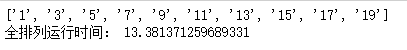10个数花了13秒的时间，可见前20个奇数所用时间非常的长，然而现在并没有找到更好地优化解决方案，只能实现到这个地步，感到非常抱歉。

问题3，实现二分查找。

在 1 3 3 4 5 5 7 8 8 9 10 中查找得到7，返回其所在的位置。
随机生成10000个整数，查找数字 2025，返回其所在的位置。
原理：

折半查找法也称为二分查找法，它充分利用了元素间的次序关系，采用分治策略，可在最坏的情况下用O(log n)完成搜索任务。它的基本思想是，将n个元素分成个数大致相同的两半，取a[n/2]与欲查找的x作比较，如果x=a[n/2]则找到x，算法终止。如 果x<a[n/2]，则我们只要在数组a的左半部继续搜索x（这里假设数组元素呈升序排列）。如果x>a[n/2]，则我们只要在数组a的右半部继续搜索x。

要求：

1.必须采用顺序存储结构。

2.必须按关键字大小有序排列。

代码实现：

# 实现二分查找。
# a) 在 1 3 3 4 5 5 7 8 8 9 10 中查找得到7，返回其所在的位置。
# b) 随机生成10000个整数，查找数字 2025，返回其所在的位置。
import random
# 二分查找，如果在lis中找到n，则返回n的下标，如果找不到，则返回-1
def binary_search(lis, n):
length = len(lis)

left = 0
right = length-1
while left <= right:
mid = (left+right)//2
# 注意，注意，注意
# 这里要注意，py3使用//作为整除，好像程老师的书《算法分析与设计》P69页存在这个错误
if lis[mid] == n:
return mid
elif lis[mid] > n:
right = mid-1
elif lis[mid] < n:
left = mid+1
return -1

if __name__ == '__main__':
lis =  [1, 3, 3, 4, 5, 5, 7, 8, 8, 9, 10]
print('数字7的下标为:',binary_search(lis, 7))

# 随机生成10000个成递增的数据
x = 0
lis2 = []
for i in range(10000):
lis2.append(x)
x += int(random.random()*10)

find = binary_search(lis2, 2026)
if find == -1:
print('没有找到2026！')
else:
print('找到2026，位置为', find)


多次运行情况：

运行one运行two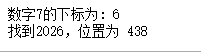问题4，实现合并排序和快速排序，比较算法的性能。

输入数据为：3 2 5 7 8 9 5 0 1

随机生成10000个整数。

实现归并排序还有快速排序

归并排序的实现方式还是记得的，写起来也毫无压力，关于快速排序这个，以前用python写过两遍，可惜的是……全部在边界数据上失败了……快速排序果然还是有点难度的，就记得个大概的方法，所以这次的快速排序是照着我一起用C++写的版本写出来的，重新用python实现了一遍。

# 实现合并排序和快速排序，比较算法的性能。
# a) 输入数据为：3 2 5 7 8 9 5 0 1
# b) 随机生成10000个整数。
import numpy as np
import random
import time

# 将两个有序列表合并，归并排序的辅助函数
def merge(lis1, lis2):
i = 0
j = 0
lis3 = [] # 合并之后的列表
while i < len(lis1) and j < len(lis2):
if lis1[i] < lis2[j]:
lis3.append(lis1[i])
i += 1
else:
lis3.append(lis2[j])
j += 1
# 处理剩下的数据
while i < len(lis1):
lis3.append(lis1[i])
i += 1
while j < len(lis2):
lis3.append(lis2[j])
j += 1
return lis3

# 归并排序 分解列表操作
def merge_sort(lis):
if len(lis) <= 1:
return lis
middle = len(lis)//2

leftlis = lis[:middle]
rightlis = lis[middle: ]
left_sorted = merge_sort(leftlis)
right_sorted = merge_sort(rightlis)
return merge(left_sorted, right_sorted)

# 快速排序，划分操作， 快速排序的辅助函数
def split(lis, first, last):
pivot = lis[first]

left = first
right = last
while left<right:
while pivot < lis[right]:
right=right-1
while left < right and (lis[left] < pivot or lis[left] == pivot):
left=left+1
if left < right:
lis[left], lis[right] = lis[right], lis[left]
# 确定好基准位置
pos = right
lis[first] = lis[pos]
lis[pos] = pivot
return pos

# 快速排序实现
def quicksort(lis, first, last):
if first < last:
pos = split(lis, first, last)
quicksort(lis, first, pos-1)
quicksort(lis, pos+1, last)
return lis

if __name__ == '__main__':
lis1 = [3, 2, 5, 7, 8, 9, 5, 0, 1]
lis2 = [3, 2, 5, 7, 8, 9, 5, 0, 1]
print('使用归并排序:',merge_sort(lis))
print('使用快速排序:',quicksort(lis, 0, len(lis)-1))

# 生成两个一样的10000个随机数的列表, 使用deepcopy函数
lis1 = [ int(random.random()*10000) for i in range(10000)]
import copy
lis2 = copy.deepcopy(lis1)	# 深度拷贝

# 测试运行时间
start = time.time()
#     print(merge_sort(lis1))  # 打印排序的数据
merge_sort(lis1)
end = time.time()
print('使用快速排序，排10000个随机数的时间:', end-start)

start = time.time()
#     print(quicksort(lis2, 0, len(lis2)-1)) # 打印排序的数据
quicksort(lis2, 0, len(lis2)-1)
end = time.time()
print('使用快速排序，排10000个随机数的时间:', end-start)



运行的结果：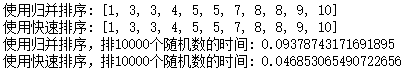这样看来，果然还是快速排序排的比较快呀~~~

三、实验总结

这次的实验内容以前全部用C++写过，现在代码还留着，所以这次使用了python语言全部重写一遍，感觉收获还是很多的，不仅使我重新巩固了有关算法方面的知识，还学会了很多新的知识和python技巧，比如优化递归的尾递归优化，py的cProfile性能分析还有itertools迭代工具模块等等，我再次深刻体会到学习算法是一件事情，把算法实现写出又是另外一件事情，也许某个算法的道理很简单，但是实现和编程过程还是需要很多的技巧的，看着写实验报告这么辛苦，于是决定把它放在博客上，就是这样。
展开全文算法
• /usr/bin/env python2 2 # -*- coding: utf-8 -*- 3 """ 4 Created on Tue Nov 21 22:28:09 2017 5 6 @author: livermorium116 7 """ 8 9 10 11 12 import random 13 14 class Me...

1 #!/usr/bin/env python2
2 # -*- coding: utf-8 -*-
3 """
4 Created on Tue Nov 21 22:28:09 2017
5
6 @author: livermorium116
7 """
8
9
10
11
12 import random
13
14 class MergeSortedByCur():
15     def __init__(self, data):
16         self.data = data
17         #print("ORIGIN DATA:",  self.data)
18         self.CountCur=0
19         self.Results=self.MergeSort(self.data)
20     def MergeSort(self,data):
21
22         if len(data) <= 1:
23                 return data
24         num = int(len(data)/2)
25         Left = self.MergeSort(data[:num])
26         Right = self.MergeSort(data[num:])
27         #print("LEFT:",Left,"RIGHT:",Right)
28         self.CountCur += 1
29         #print ("%d Cursions:"%(self.CountCur))
30         return self.Merge(Left, Right)
31
32
33     def Merge(self,left, right):
34         R, L = 0, 0
35         result = []
36         while L < len(left ) and R < len(right ):
37             if left[L] < right[R]:
38                 result.append(left[L])
39                 L += 1
40             else:
41                 result.append(right[R])
42                 R += 1
43         result.extend(left[L:])
44         result.extend(right[R:])
45         #print("MergeSorted DATA:",result)
46         return result
47
48
49
50
51
52
53
54
55 if __name__ == "__main__":
56     data = [random.randint(1,100) for i in range(13)]
57     print(data)
58     print MergeSortedByCur(data).Results
59

转载于:https://www.cnblogs.com/milliard/p/7875881.html
展开全文• 所谓归并操作，是指将两个已经排序好的序列合并成一个序列的操作。因此，基于分治法的思想和归并操作，归并排序的具体步骤如下。 递归法步骤： 将 待排序序列分成两个子序列，申请两个大小为两个子序列大小的空间，...算法
• 剑指offer：合并两个排序的链表，Python实现 题目描述 输入两个单调递增的链表，输出两个链表合成后的链表，当然我们需要合成后的链表满足单调不减规则。 吐槽 本来想用递归实现，但是大脑卡壳，没有想到合适的递归...
• 上一篇中，我们实现了一般的归并排序 归并排序递归与非递归-Python实现 在实际工作中，多个有序数列合并成一个，大文件或多个大文件合并成一个并排序的需求常见并不少见，首先，先来看一下多个有序数列情况 合并多个...数据结构 大文件
• 有序序列开始可能为0，在每一次操作（循环或者递归）后，有序序列数目会加1，无序序列数目会减一，代码走完后，原本的数据序列完全变成有序序列。 在学校常写的简单排序，确实是简单粗暴。 def sort(lyst): for ...
• 2019独角兽企业重金招聘Python工程师标准>>> ...
• 剑指offer刷题笔记16（Python） 题目描述 输入两个单调递增的链表，输出两个链表合成后的链表，当然我们需要合成后的链表满足单调不减规则。 思路1 利用递归 代码1 class Solution: # 返回合并后列表 def Merge...
• 题目描述 输入两个单调递增的链表，输出两个链表合成后的链表，当然我们需要...递归 class Solution: # 返回合并后列表 def Merge(self, pHead1, pHead2): # write code here if pHead1 == None: return pHead2...
• 如果列表有多个项，我们分割列表，并递归调用两个半部分的合并排序。 一旦对这两半排序完成，就执行称为合并的基本操作。合并是获取两个较小的排序列表并将它们组合成单个排序的新列表的过程。 def mergeSort(alist)...
• ##1.4 题17 合并两个排序的链表 ##题目：输入两个单调递增的链表，输出两个链表合成后的链表，当然我们需要合成后的链表蛮族单调不减规则 #关于链表： # class ListNode: # def __init__(self, x): # self....
• 递归 原理比较简单，就是有序数组的合并。 def merge(a, b): c = [] i = j = 0 while i < len(a) and j < len(b): if a[i] < b[j]: c.append(a[i]) i += 1 else: ...
• 剑指offer（十六）合并两个排序的链表 递归方法递归代码递归详解 最近刷题发现我真是个菜鸡，看书人家都说这道题hen简单我们这样这样就好然后直接贴代码，奈何我这样都看不懂，尤其是有的题的递归解法，我全是啥玩意...剑指offer
• 合并两个排序好的链表”思路有很多种，推荐用递归数据结构与算法 链表
• python 归并排序递归法与循环法(利用队列)实现，以及性能测试 递归排序核心 递归排序的核心是 分与合 分的最终结果 就是将原数组中每一个数字分作一个数组， 合就是 所有小数组不断排序合并的过程。 合并的过程...
• 网络上用python实现快速排序有四种实现方式，有用匿名函数lambda表达式和双重循环实现的，也有用栈实现非递归排序，这里我只讲一讲利用算法导论里面的分治思想，迭代来实现序列的快速排序。分治策略是对于一个规模...经典算法
• 思想：先递归分解数组，再合并数组 原理：将数组分解最小之后，然后合并两个有序数组，基本思想是比较两个数组的最前面的数，谁小就取谁，取完后，将相应的指针后移以为。然后再比较，直到一个数组为空，最后把另一...
• 归并排序 时间复杂度O(nlogn) 空间复杂度O(n) ...合并：将两个有序的列表合并，列表越来越大 代码实现 # 归并排序 import random import sys sys.setrecursionlimit(10000) # 设置递归深度默认1000 # ...
• 如果列表有多个项，我们分割列表，并递归调用两个半部分的合并排序。 一旦对这两半排序完成，就执行称为合并的基本操作。 合并是获取两个较小的排序列表并将它们组合成单个排序的新列表的过程。 代码： def ...
• 归并排序也称合并排序，是分治法的典型应用。分治思想是将每个问题分解成个个小问题，将每个小问题解决，然后合并。 具体的归并排序就是，将一组无序数按n/2递归分解成只有一个元素的子项，一个元素就是已经排好序的...算法
• 运用递归的思想到合并排序。 我一直对递归思想怀有“敬畏”。O(∩_∩)O，至少在写代码时时很简洁、漂亮的。 只是大概明白合并排序的流程，导致编程时出现了思绪不清。递归不知道怎么写。 A = [8,2,6,1,4,5,7,3,0...
• 解决Conquer：递归合并排序法对2个子序列递归的排序 3.合并Combine:合并2个已排序的子序列以得到排序结果 */ #include #include using namespace std; #define N 8 #define MAX 100 //哨兵
• #合并两个递增的链表并使新链表依旧按照递增排序--递归实现 def mergeLinkedList(pHead1, pHead2): if pHead1 is None: return pHead2 elif pHead2 is None: return pHead1 pMergeHead = None if pHead......

# python递归合并排序python 订阅